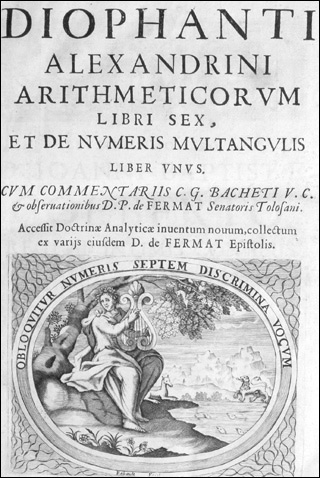18.782 | Fall 2013 | Undergraduate

# Introduction to Arithmetic Geometry

#### Course Description

This course is an introduction to arithmetic geometry, a subject that lies at the intersection of algebraic geometry and number theory. Its primary motivation is the study of classical Diophantine problems from the modern perspective of algebraic geometry.

## Course Info

##### Learning Resource Types
Problem Sets
Lecture Notes
Online Textbook
Instructor InsightsFrontispiece of the 1670 edition of Diophantus’ Arithmetica. The 1670 edition reproduces notes and observations that Pierre de Fermat wrote in the margins of the 1621 first printed edition. (This image is in the public domain.)